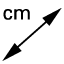# Proposition I.1 Construction

Author:
Eric Haney

## Construct an equilateral triangle

Use the tools below to construct an equilateral triangle following the construction steps of Proposition I.1. When you have completed the equilateral triangle, click each side of the triangle with theDistance tool to measure each of the sides.

Which postulates were used in the construction? Select all that apply.

Select all that apply
• A
• B
• C
• D

According to the definitions, what is the name of a triangle that has only two equal sides?

Select all that apply
• A
• B
• C